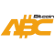Bitcoin ABC  0.22.12 P2P Digital Currency
coinselection.cpp File Reference
`#include <wallet/coinselection.h>`
`#include <util/moneystr.h>`
`#include <util/system.h>`
Include dependency graph for coinselection.cpp:

Go to the source code of this file.

## Functions

bool SelectCoinsBnB (std::vector< OutputGroup > &utxo_pool, const Amount &target_value, const Amount &cost_of_change, std::set< CInputCoin > &out_set, Amount &value_ret, const Amount not_input_fees)

static void ApproximateBestSubset (const std::vector< OutputGroup > &groups, const Amount &nTotalLower, const Amount &nTargetValue, std::vector< char > &vfBest, Amount &nBest, int iterations=1000)

bool KnapsackSolver (const Amount nTargetValue, std::vector< OutputGroup > &groups, std::set< CInputCoin > &setCoinsRet, Amount &nValueRet)

## Variables

struct {
descending

static const size_t TOTAL_TRIES = 100000
This is the Branch and Bound Coin Selection algorithm designed by Murch. More...

## ◆ ApproximateBestSubset()

 static void ApproximateBestSubset ( const std::vector< OutputGroup > & groups, const Amount & nTotalLower, const Amount & nTargetValue, std::vector< char > & vfBest, Amount & nBest, int iterations = `1000` )
static

Definition at line 210 of file coinselection.cpp.

Here is the call graph for this function:
Here is the caller graph for this function:

## ◆ KnapsackSolver()

 bool KnapsackSolver ( const Amount nTargetValue, std::vector< OutputGroup > & groups, std::set< CInputCoin > & setCoinsRet, Amount & nValueRet )

Definition at line 252 of file coinselection.cpp.

Here is the call graph for this function:
Here is the caller graph for this function:

## ◆ SelectCoinsBnB()

 bool SelectCoinsBnB ( std::vector< OutputGroup > & utxo_pool, const Amount & target_value, const Amount & cost_of_change, std::set< CInputCoin > & out_set, Amount & value_ret, const Amount not_input_fees )

Definition at line 69 of file coinselection.cpp.

Here is the call graph for this function:
Here is the caller graph for this function:

## ◆ descending

 struct { ... } descending

## ◆ TOTAL_TRIES

 const size_t TOTAL_TRIES = 100000
static

This is the Branch and Bound Coin Selection algorithm designed by Murch.

It searches for an input set that can pay for the spending target and does not exceed the spending target by more than the cost of creating and spending a change output. The algorithm uses a depth-first search on a binary tree. In the binary tree, each node corresponds to the inclusion or the omission of a UTXO. UTXOs are sorted by their effective values and the trees is explored deterministically per the inclusion branch first. At each node, the algorithm checks whether the selection is within the target range. While the selection has not reached the target range, more UTXOs are included. When a selection's value exceeds the target range, the complete subtree deriving from this selection can be omitted. At that point, the last included UTXO is deselected and the corresponding omission branch explored instead. The search ends after the complete tree has been searched or after a limited number of tries.

The search continues to search for better solutions after one solution has been found. The best solution is chosen by minimizing the waste metric. The waste metric is defined as the cost to spend the current inputs at the given fee rate minus the long term expected cost to spend the inputs, plus the amount the selection exceeds the spending target:

waste = selectionTotal - target + inputs × (currentFeeRate - longTermFeeRate)

The algorithm uses two additional optimizations. A lookahead keeps track of the total value of the unexplored UTXOs. A subtree is not explored if the lookahead indicates that the target range cannot be reached. Further, it is unnecessary to test equivalent combinations. This allows us to skip testing the inclusion of UTXOs that match the effective value and waste of an omitted predecessor.

The Branch and Bound algorithm is described in detail in Murch's Master Thesis: https://murch.one/wp-content/uploads/2016/11/erhardt2016coinselection.pdf

Parameters
 const std::vector& utxo_pool The set of UTXOs that we are choosing from. These UTXOs will be sorted in descending order by effective value and the CInputCoins' values are their effective values. const Amount& target_value This is the value that we want to select. It is the lower bound of the range. const Amount& cost_of_change This is the cost of creating and spending a change output. This plus target_value is the upper bound of the range. std::set& out_set -> This is an output parameter for the set of CInputCoins that have been selected. Amount& value_ret -> This is an output parameter for the total value of the CInputCoins that were selected. Amount not_input_fees -> The fees that need to be paid for the outputs and fixed size overhead (version, locktime, marker and flag)

Definition at line 67 of file coinselection.cpp.